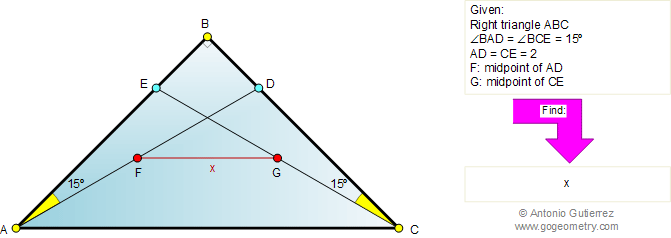Problem 406. Right triangle, 15 degrees, Midpoints, Congruence. Level: High School, College, SAT Prep. The figure shows a right triangle ABC with AD = CE = 2. The measure of angles BAD and BCE are equal to 15 degrees. If F and G are the midpoints of AD and CE, respectively, find FG.Recent Additions